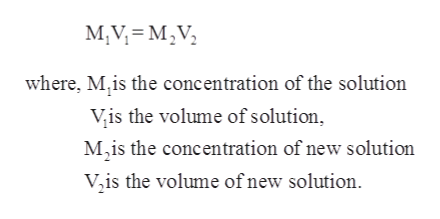# How would you prepare 10 mL of a 0.25% m/v HCl solution if 1% m/v HCl was available? How much 1% m/v HCl is needed? How much distilled water is used?

Question
62 views

How would you prepare 10 mL of a 0.25% m/v HCl solution if 1% m/v HCl was available? How much 1% m/v HCl is needed? How much distilled water is used?

check_circle

Step 1

It is given that:

Volume (V1) of solution = 10 mL

Concentration of HCl solution (M1) = 0.25 %

Concentration of available solution of HCl (M2) = 1 %

Step 2

Law of equivalence states that the volume of titrants reacted have same number of equivalents points at the end point of titration. The expression for this is given as follows:help_outlineImage TranscriptioncloseM,V MV where, M,is the concentration of the solution Vis the volume of solution M2is the concentration of new solution V,is the volume of new solution fullscreen
Step 3

Now using law of equivalence equation, the volume o...

### Want to see the full answer?

See Solution

#### Want to see this answer and more?

Solutions are written by subject experts who are available 24/7. Questions are typically answered within 1 hour.*

See Solution
*Response times may vary by subject and question.
Tagged in

### Chemistry